Create a new printable
Answer key also includes questionsRatios
Math Worksheets

Sample - Click above to make a new math worksheet (PDF).
 Name _____________________________Date ___________________
Ratios
Use a proportion to find the unknown length in the pair of similar figures.
(drawings are not drawn to scale)

1.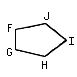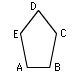length of sides:
 HG  = 72 cm IH  = 54 cm FJ  = 24 cm GF  = 66 cm JI  = 36 cm

 CB  = 144 cm AE  = 48 cm DC  = 108 cm BA  = 132 cm ED  = ________
2.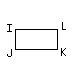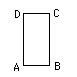length of sides:
 LI  = 14 m JK  = ________ KL  = ________ IJ  = 70 m

 CD  = 100 m DA  = 20 m BC  = 20 m AB  = 100 m
3.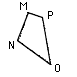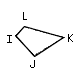length of sides:
 PM  = 78 ft NO  = 84 ft MN  = 48 ft OP  = ________

 LK  = 15 ft IL  = 70 ft JI  = 40 ft KJ  = 65 ft
4.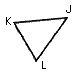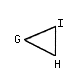length of sides:
 JL  = 30 yd KJ  = 72 yd KL  = 36 yd

 IH  = ________ GI  = ________ GH  = 12 yd
 5 * This is a pre-made sheet.Use the link at the top of the page for a printable page.
6.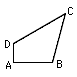length of sides:
 JI  = ________ LK  = 3 km IL  = 14 km KJ  = 13 km

 CB  = 104 km AD  = 112 km DC  = 24 km BA  = 16 km